# Probability – 1 – 3

11. If A & B are any two events, then the true relation is :

(a) P (A ∩B) > P (A) + P (B) – 1
(b) P (A ∩B) < P (A) + P (B)
(c) P (A ∩ B) = P (A) + P (B) − P (A ∪ B)
(d) none of these

Explanation
No answer description available for this question. Let us discuss.

12. A purse contains 4 copper coins & 3 silver coins, the second purse contains 6 copper coins & 2 silver coins. If a coin is drawn out of any purse, then the probability that it is a copper coin, is :

(a) 4/7
(b) 3/4
(c) 37/56
(d) none of these

Explanation
No answer description available for this question. Let us discuss.

13. If A & B are any two events, then the probability that exactly one of them occur is :

(a) P (A) + P (B) – P (A ∩B)
(b) P (A) + P (B) – 2 P (A ∩B)
(c) P (A) + P (B) – P (A ∪B)
(d) P (A) + P (B) – 2 P (A ∪B)

Explanation
No answer description available for this question. Let us discuss.

14. If A & B are two mutually exclusive events, then P (A + B) =

(a) P (A) + P (B) – P (AB)
(b) P (A) – P (B)
(c) P (A) + P (B)
(d) P (A) + P (B) + P (AB)

Explanation
No answer description available for this question. Let us discuss.

15. The probability of happening atleast one of the events A & B is 0.6. If the events A & B happens simultaneously with the probability 0.2, then(a) 0.4
(b) 0.8
(c) 1.2
(d) none of these

Explanation
No answer description available for this question. Let us discuss.

General Knowledge Books##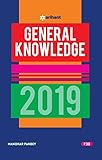General Knowledge 2019 Price: Check on Amazon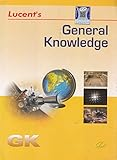-17% General Knowledge Price: INR 179.00 Was: INR 216.00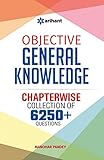-43% Objective General Knowledge Chapterwise Collection of 6250+ Questions Price: INR 169.00 Was: INR 295.00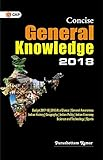Concise General Knowledge 2018 Price: INR 30.00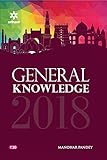General Knowledge 2018 (Old Edition) Price: Check on Amazon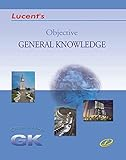-16% Objective General Knowledge Price: INR 302.00 Was: INR 360.00 ‹›

Be a Part of the New & Next

Share the Knowledge

Copy Protected by Chetan's WP-Copyprotect.